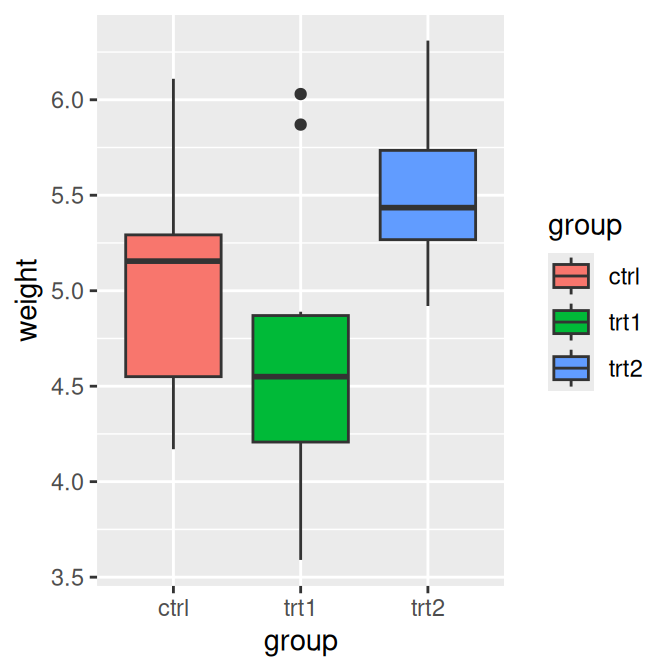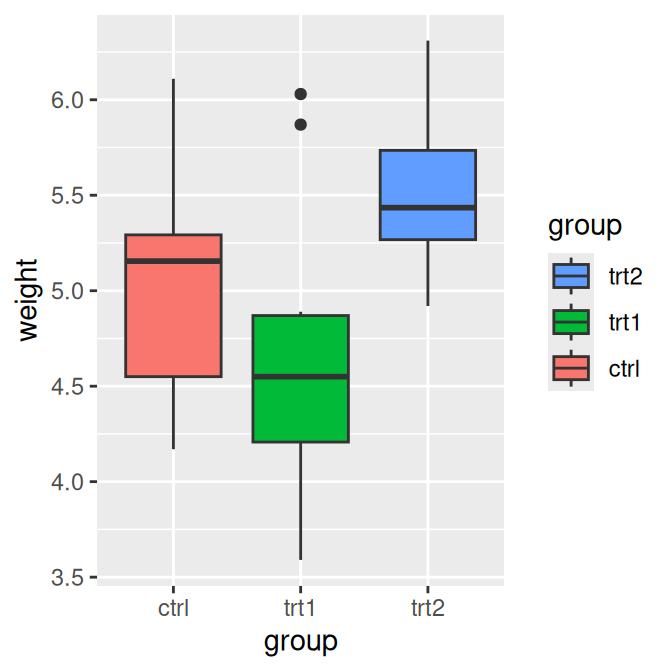## 10.4 Reversing the Order of Items in a Legend

### 10.4.1 Problem

You want to reverse the order of items in a legend.

### 10.4.2 Solution

Add guides (`fill = guide_legend(reverse = TRUE)`) to reverse the order of the legend, as in Figure 10.7 (for other aesthetics, replace `fill` with the name of the aesthetic, such as `colour` or `size`):

``````# Create the base plot
pg_plot <- ggplot(PlantGrowth, aes(x = group, y = weight, fill = group)) +
geom_boxplot()

pg_plot

# Reverse the legend order
pg_plot +
guides(fill = guide_legend(reverse = TRUE))``````Figure 10.7: Default order for legend (left); Reversed order (right)

### 10.4.3 Discussion

It is also possible to control the legend when specifying the scale, as in the following:

``scale_fill_hue(guide = guide_legend(reverse = TRUE))``